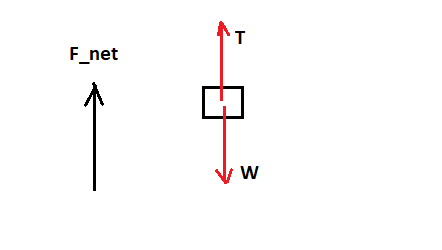# A 1000 kg elevator accelerates upward at 1 m/s for 10 m, starting from rest. a. How much work...

## Question:

A 1000 kg elevator accelerates upward at 1 m/s for 10 m, starting from rest.

a. How much work does gravity do on the elevator? J

b. How much work does the tension in the elevator cable do on the elevator? J

c. Use the work-kinetic energy theorem to find the kinetic energy of the elevator as it reaches 10 m. J

d. What is the speed of the elevator as it reaches 10 m? m/s

## Work-Kinetic Energy Theorem:

The work-kinetic energy theorem states that the work done on an object by a net force acting on it is equal to the change in the kinetic energy of the object. This means that if the net force does negative work, the object loses kinetic energy and if it does positive work, the object gains kinetic energy.

In our case, we have an elevator of mass {eq}m \ = \ 1000 \ kg {/eq} accelerating upwards at {eq}a \ = \ 1 \ m/s^2 {/eq}. From Newton's second law of motion, the net force acting on the elevator is

{eq}F_{net} \ = \ m \ a \ = \ 1000 \ kg \ \times \ 1 \ m/s^2 \ = \ 1000 \ N {/eq}

directed upwards. The weight of the elevator is

{eq}W \ = \ 1000 \ kg \ \times \ 9.81 \ m/s^2 \ = \ 9810 \ N {/eq}

There are two forces acting on the elevator as it moves. Theses are the weight and the tension T as shown in the image below.Therefore, the tension in the elevator rope is

{eq}T \ = \ F_{net} \ + \ W \ = \ 1000 \ N \ + \ 9810 \ N \ = \ 10810 \ N {/eq}

The distance moved by the elevator is given to be {eq}d \ = \ 10 \ m {/eq}. The work done by a force F in moving an object a distance d is given by

{eq}\mathrm{Work} \ = \ F \ d \ \cos \theta {/eq}

where {eq}\theta {/eq} is the angle between the direction of the force and the direction of motion of the object.

a: The work done by gravity (or the weight) is

{eq}\mathrm{Work}_g \ = \ W \ d \ \cos 180^{\circ} \ = \ - \ 9810 \ N \ \times \ 10 \ m \ = \ \mathbf{- \ 9.81 \ \times \ 10^4 \ J} {/eq}

b: The work done by the tension in the cable is

{eq}\mathrm{Work}_T \ = \ T \ d \ \cos 0^{\circ} \ = \ 10810 \ N \ \times \ 10 \ m \ = \ \mathbf{1.081 \ \times \ 10^5 \ J} {/eq}

c: The net work done on the elevator is given hy the sum of the work done by the tension and gravity.

{eq}\mathrm{Work} \ = \ - \ 9.81 \ \times \ 10^4 \ J \ + \ 1.081 \ \times \ 10^5 \ J \ = \ 1.00 \ \times \ 10^4 \ J {/eq}

The work-kinetic energy theorem states that the work done on an object by a net force acting on it is equal to the change in the kinetic energy of the object. Assuming the elevator started from rest, its kinetic energy as it reaches 10 m is {eq}\mathbf{1.00 \ \times \ 10^4 \ J} {/eq}.

d: This kinetic energy is given by

{eq}K.E \ = \ 1.00 \ \times \ 10^4 \ J \ = \ 0.5 \ m \ v^2 {/eq}

where v is the speed of the elevator as it reaches 10. Solving for v, we get

{eq}v \ = \ \sqrt{\dfrac{2 \ \times \ 1.00 \ \times \ 10^4 \ J}{1000 \ kg}} \ = \ \mathbf{4.47 \ m/s} {/eq}

correct to three significant figures.